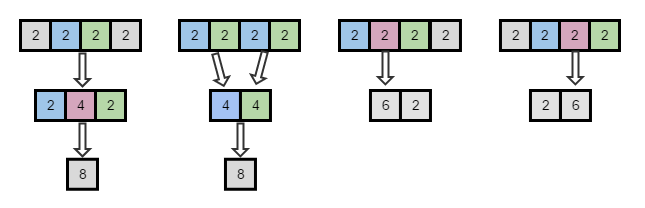2016-S4 Combining Rice Balls

# 2016-S4 Combining Rice Balls

Problem Description
View Problem

## Analysis

At first glance, this problem appears to require a decision tree on when combinations will take place.

Take the following example:

 2 2 2 2

In the examples below we look for the following areas that allow us to combine balls;

 Start Block Middle Block End Block

It can be combined in the following ways:## Key Insight

With the exception of the dead end solutions, the first two result in a single ball of size 8. The way that we got to the single ball is no longer important because we now know that this range of balls can be combined and the size of the resulting ball will always be the sum of all the balls. This allows us to reduce what would have been 4 potential options (there is a mirror to approach #2) into a single answer and this allows us to use dynamic programming to remember that answer efficiently.

## Algorithm

The solution is to combine all possible rice balls and find the largest one. The way to do this efficiently, however, is to reduce the amount of work required by keeping a record of the balls that can be combined. This approach is Dynamic Programming and is the key algorithm that makes these solutions possible quickly.

### Search Pattern

To search for a valid solution we must start with attempting to combine 2 adjacent balls at a time. Once we have found all 2 ball solutions we attempt to identify areas with 3 balls that can be combined. This process continues until we determine if all the balls can be combined.

If these 3 areas can each be combined into a single ball (the middle block is optional) and the total ball count of the Start Block equals the total ball count of the End block, this entire section can be combined. All search patterns will be identified by the following syntax:

``````Start(StartIndex,EndIndex) Middle(StartIndex,EndIndex) End(StartIndex,EndIndex)
``````

Searching for 2 ball combinations using the start/end block approach:

 0 1 2 3 Search Result 2 2 2 2 S(0) E(1) YES (4) 2 2 2 2 S(1) E(2) YES (4) 2 2 2 2 S(2) E(3) YES (4)

Searching for 3 ball combinations using the start/end block approach:

 0 1 2 3 Search Result Why Answers Used 2 2 2 2 S(0) E(1,2) NO 2 != 4 (1,2) 2 2 2 2 S(0,1) E(2) NO 4 != 2 (0,1) 2 2 2 2 S(1) E(2,3) NO 2 != 4 (2,3) 2 2 2 2 S(1,2) E(3) NO 4 != 2 (1,2)

Searching for 3 ball combinations using the start/middle/end block approach:

 0 1 2 3 Search Result Why Answers Used 2 2 2 2 S(0) M(1) E(2) YES (6) 2 = 2 2 2 2 2 S(1) M(2) E(3) YES (6) 2 = 2

Searching for 4 ball combinations using the start/end block approach:

 0 1 2 3 Search Result Why Answers Used 2 2 2 2 S(0) E(1,3) NO 2 != 6 (1,3) 2 2 2 2 S(1,2) E(2,3) YES (8) 4 = 4 (0,1) & (2,3) 2 2 2 2 S(0,2) E(3) NO 6 != 2 (0,2)

Searching for 4 ball combinations using the start/middle/end block approach:

 0 1 2 3 Search Result Why Answers Used 2 2 2 2 S(0) E(1,3) NO 2 != 4 (2,3) 2 2 2 2 S(1,2) E(2,3) YES (8) 2 = 2 (1,2) 2 2 2 2 S(0,2) E(3) NO 4 != 2 (0,1)

As you can see even a simple problem of 4 rice balls has a substantial number of permutations to look through and it is important that we can quickly rule out a large number of checks. In many cases a check can be quickly removed if an answer for a range cannot be found. Also, as soon as a single valid answer is found for the range in question we can stop - we don’t need to find multiple answers as they produce the identical result.

## Dynamic Programming

In this case, it is clear that the best choice of DP container is a 2-dimensional array because each range being stored contains a start and end index. Other containers like maps or dictionaries could be used but would have much lower performance.

Please attempt to solve the problem on your own before viewing the solution!
View Solution